# 1095 Results

View
Selected filters:
• Illustrative Mathematics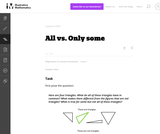Unrestricted Use
CC BY
Rating

This is a task from the Illustrative Mathematics website that is one part of a complete illustration of the standard to which it is aligned. Each task has at least one solution and some commentary that addresses important asects of the task and its potential use. Here are the first few lines of the commentary for this task: First pose the question: Here are four triangles. What do all of these triangles have in common? What makes them different from the figures that are no...

Subject:
Elementary Mathematics
Material Type:
Activity/Lab
Provider:
Illustrative Mathematics
Provider Set:
Illustrative Mathematics
Author:
Illustrative Mathematics
05/24/2013Unrestricted Use
CC BY
Rating

This is a task from the Illustrative Mathematics website that is one part of a complete illustration of the standard to which it is aligned. Each task has at least one solution and some commentary that addresses important asects of the task and its potential use. Here are the first few lines of the commentary for this task: Materials * A copy of Grandfather Tang's Story by Ann Tompert * One set of tangrams for each student (see note in commentary) * A set of tangrams for t...

Subject:
Elementary Mathematics
Material Type:
Activity/Lab
Provider:
Illustrative Mathematics
Provider Set:
Illustrative Mathematics
Author:
Illustrative Mathematics
05/29/2013Unrestricted Use
CC BY
Rating

This is a task from the Illustrative Mathematics website that is one part of a complete illustration of the standard to which it is aligned. Each task has at least one solution and some commentary that addresses important asects of the task and its potential use. Here are the first few lines of the commentary for this task: Materials - 2 clear plastic cups for each pair of students - 4 bean seeds for each pair - soil - unifix cubes - a plant or math journal to record data ...

Subject:
Elementary Mathematics
Material Type:
Activity/Lab
Provider:
Illustrative Mathematics
Provider Set:
Illustrative Mathematics
Author:
Illustrative Mathematics
04/17/2013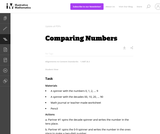Unrestricted Use
CC BY
Rating

This is a task from the Illustrative Mathematics website that is one part of a complete illustration of the standard to which it is aligned. Each task has at least one solution and some commentary that addresses important asects of the task and its potential use. Here are the first few lines of the commentary for this task: Materials A spinner with the numbers 0, 1, 2, ... 9 A spinner with the decades 00, 10, 20, ... 90 Math journal or teacher-made worksheet Pencil Actions...

Subject:
Elementary Mathematics
Material Type:
Activity/Lab
Provider:
Illustrative Mathematics
Provider Set:
Illustrative Mathematics
Author:
Illustrative Mathematics
04/19/2013Unrestricted Use
CC BY
Rating

This is a task from the Illustrative Mathematics website that is one part of a complete illustration of the standard to which it is aligned. Each task has at least one solution and some commentary that addresses important aspects of the task and its potential use.

Subject:
Mathematics
Material Type:
Activity/Lab
Provider:
Illustrative Mathematics
Provider Set:
Illustrative Mathematics
Author:
Illustrative Mathematics
07/02/2018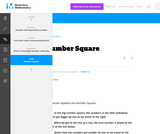Unrestricted Use
CC BY
Rating

This is a task from the Illustrative Mathematics website that is one part of a complete illustration of the standard to which it is aligned. Each task has at least one solution and some commentary that addresses important aspects of the task and its potential use.

Subject:
Mathematics
Material Type:
Activity/Lab
Provider:
Illustrative Mathematics
Provider Set:
Illustrative Mathematics
Author:
Illustrative Mathematics
07/02/2018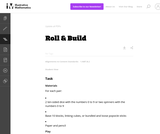Unrestricted Use
CC BY
Rating

This is a task from the Illustrative Mathematics website that is one part of a complete illustration of the standard to which it is aligned. Each task has at least one solution and some commentary that addresses important asects of the task and its potential use. Here are the first few lines of the commentary for this task: Materials For each pair: * 2 ten-sided dice with the numbers 0 to 9 or two spinners with the numbers 0 to 9 * Base-10 blocks, linking cubes, or bundled...

Subject:
Elementary Mathematics
Material Type:
Activity/Lab
Provider:
Illustrative Mathematics
Provider Set:
Illustrative Mathematics
Author:
Illustrative Mathematics
05/24/2013Unrestricted Use
CC BY
Rating

This is a task from the Illustrative Mathematics website that is one part of a complete illustration of the standard to which it is aligned. Each task has at least one solution and some commentary that addresses important asects of the task and its potential use. Here are the first few lines of the commentary for this task: Materials Set of cards from 1-100, these can be purchased or created using a black marker and index cards Setup Sort the 1-100 cards into sequential gr...

Subject:
Elementary Mathematics
Material Type:
Activity/Lab
Provider:
Illustrative Mathematics
Provider Set:
Illustrative Mathematics
Author:
Illustrative Mathematics
03/16/2013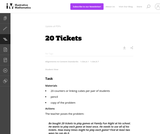Unrestricted Use
CC BY
Rating

This is a task from the Illustrative Mathematics website that is one part of a complete illustration of the standard to which it is aligned. Each task has at least one solution and some commentary that addresses important asects of the task and its potential use. Here are the first few lines of the commentary for this task: Materials 20 counters or linking cubes per pair of students pencil copy of the problem Actions The teacher poses the problem: Bo bought 20 tickets to p...

Subject:
Elementary Mathematics
Material Type:
Activity/Lab
Provider:
Illustrative Mathematics
Provider Set:
Illustrative Mathematics
Author:
Illustrative Mathematics
05/05/2013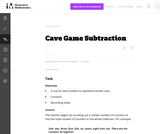Unrestricted Use
CC BY
Rating

This is a task from the Illustrative Mathematics website that is one part of a complete illustration of the standard to which it is aligned. Each task has at least one solution and some commentary that addresses important asects of the task and its potential use. Here are the first few lines of the commentary for this task: Materials * A cup for each student to represent his/her cave * Counters * Recording sheet Actions The teacher begins by counting out a certain number o...

Subject:
Elementary Mathematics
Material Type:
Activity/Lab
Provider:
Illustrative Mathematics
Provider Set:
Illustrative Mathematics
Author:
Illustrative Mathematics
03/25/2014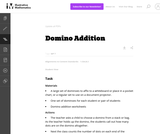Unrestricted Use
CC BY
Rating

This is a task from the Illustrative Mathematics website that is one part of a complete illustration of the standard to which it is aligned. Each task has at least one solution and some commentary that addresses important asects of the task and its potential use. Here are the first few lines of the commentary for this task: Materials A large set of dominoes to affix to a whiteboard or place in a pocket chart, or a regular set to use on a document projector. One set of domi...

Subject:
Elementary Mathematics
Material Type:
Activity/Lab
Provider:
Illustrative Mathematics
Provider Set:
Illustrative Mathematics
Author:
Illustrative Mathematics
05/24/2013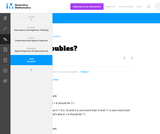Unrestricted Use
CC BY
Rating

This is a task from the Illustrative Mathematics website that is one part of a complete illustration of the standard to which it is aligned. Each task has at least one solution and some commentary that addresses important aspects of the task and its potential use.

Subject:
Mathematics
Material Type:
Activity/Lab
Provider:
Illustrative Mathematics
Provider Set:
Illustrative Mathematics
Author:
Illustrative Mathematics
07/02/2018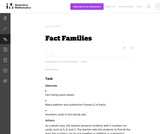Unrestricted Use
CC BY
Rating

This is a task from the Illustrative Mathematics website that is one part of a complete illustration of the standard to which it is aligned. Each task has at least one solution and some commentary that addresses important asects of the task and its potential use. Here are the first few lines of the commentary for this task: Materials * Fact Family work sheets * Blank addition and subtraction frames (2 of each) * Numbers cards in fact-family sets Actions As a whole class, t...

Subject:
Elementary Mathematics
Material Type:
Activity/Lab
Provider:
Illustrative Mathematics
Provider Set:
Illustrative Mathematics
Author:
Illustrative Mathematics
09/06/2013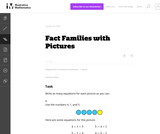Unrestricted Use
CC BY
Rating

This is a task from the Illustrative Mathematics website that is one part of a complete illustration of the standard to which it is aligned. Each task has at least one solution and some commentary that addresses important asects of the task and its potential use. Here are the first few lines of the commentary for this task: Write as many equations for each picture as you can. Use the numbers 4, 1, and 5. Here are some equations for this picture. 4+1=5 \hskip4em 5 = 4+1 5-1...

Subject:
Elementary Mathematics
Material Type:
Activity/Lab
Provider:
Illustrative Mathematics
Provider Set:
Illustrative Mathematics
Author:
Illustrative Mathematics
03/25/2014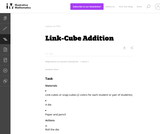Unrestricted Use
CC BY
Rating

This is a task from the Illustrative Mathematics website that is one part of a complete illustration of the standard to which it is aligned. Each task has at least one solution and some commentary that addresses important asects of the task and its potential use. Here are the first few lines of the commentary for this task: Materials * Link-cubes or snap-cubes (2 colors for each student or pair of students) * A die * Paper and pencil Actions 1. Roll the die. 2. Using a sin...

Subject:
Elementary Mathematics
Material Type:
Activity/Lab
Provider:
Illustrative Mathematics
Provider Set:
Illustrative Mathematics
Author:
Illustrative Mathematics
09/18/2013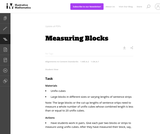Unrestricted Use
CC BY
Rating

This is a task from the Illustrative Mathematics website that is one part of a complete illustration of the standard to which it is aligned. Each task has at least one solution and some commentary that addresses important asects of the task and its potential use. Here are the first few lines of the commentary for this task: Materials * Unifix cubes * Large blocks in different sizes or varying lengths of sentence strips Note: The large blocks or the cut-up lengths of senten...

Subject:
Elementary Mathematics
Material Type:
Activity/Lab
Provider:
Illustrative Mathematics
Provider Set:
Illustrative Mathematics
Author:
Illustrative Mathematics
03/18/2014Unrestricted Use
CC BY
Rating

This is a task from the Illustrative Mathematics website that is one part of a complete illustration of the standard to which it is aligned. Each task has at least one solution and some commentary that addresses important asects of the task and its potential use. Here are the first few lines of the commentary for this task: Materials The Very Hungry Caterpillar by Eric Carle The students work individually or in pairs. Each student or pair needs: Three ten-frames for each s...

Subject:
Elementary Mathematics
Material Type:
Activity/Lab
Provider:
Illustrative Mathematics
Provider Set:
Illustrative Mathematics
Author:
Illustrative Mathematics
04/24/2013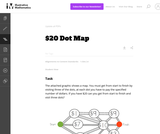Unrestricted Use
CC BY
Rating

This problem helps students practice adding three numbers whose sum are 20 or less.

Subject:
Elementary Mathematics
Material Type:
Activity/Lab
Provider:
Illustrative Mathematics
Provider Set:
Illustrative Mathematics
Author:
Illustrative Mathematics
09/10/2012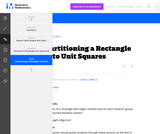Unrestricted Use
CC BY
Rating

This is a task from the Illustrative Mathematics website that is one part of a complete illustration of the standard to which it is aligned. Each task has at least one solution and some commentary that addresses important aspects of the task and its potential use.

Subject:
Mathematics
Material Type:
Activity/Lab
Provider:
Illustrative Mathematics
Provider Set:
Illustrative Mathematics
Author:
Illustrative Mathematics
07/02/2018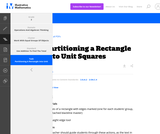Unrestricted Use
CC BY
Rating

This is a task from the Illustrative Mathematics website that is one part of a complete illustration of the standard to which it is aligned. Each task has at least one solution and some commentary that addresses important aspects of the task and its potential use.

Subject:
Mathematics
Material Type:
Activity/Lab
Provider:
Illustrative Mathematics
Provider Set:
Illustrative Mathematics
Author:
Illustrative Mathematics